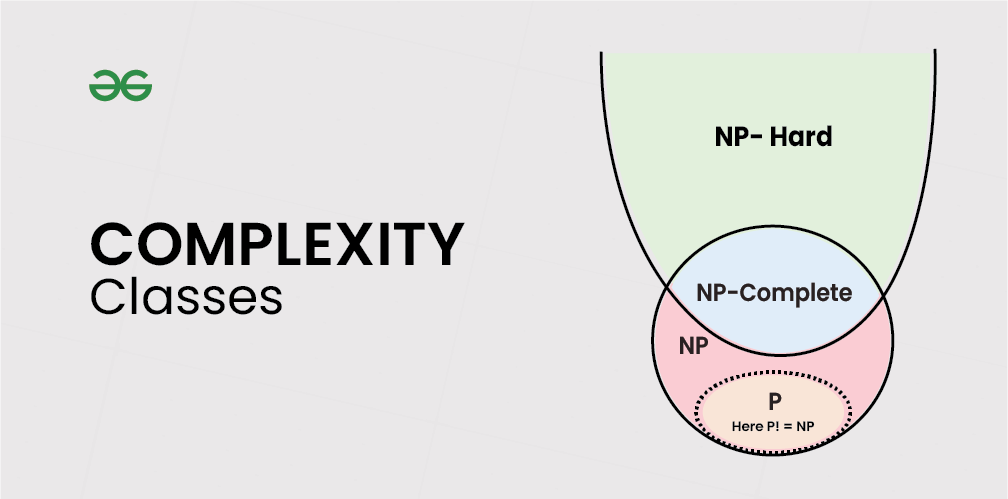# P, NP, CoNP, NP hard and NP complete | Complexity Classes

In computer science, there exist some problems whose solutions are not yet found, the problems are divided into classes known as Complexity Classes. In complexity theory, a Complexity Class is a set of problems with related complexity. These classes help scientists to group problems based on how much time and space they require to solve problems and verify the solutions. It is the branch of the theory of computation that deals with the resources required to solve a problem.

The common resources are time and space, meaning how much time the algorithm takes to solve a problem and the corresponding memory usage.

• The time complexity of an algorithm is used to describe the number of steps required to solve a problem, but it can also be used to describe how long it takes to verify the answer.
• The space complexity of an algorithm describes how much memory is required for the algorithm to operate.

Complexity classes are useful in organising similar types of problems.1. P Class
2. NP Class
3. CoNP Class
4. NP-hard
5. NP-complete

## P Class

The P in the P class stands for Polynomial Time. It is the collection of decision problems(problems with a “yes” or “no” answer) that can be solved by a deterministic machine in polynomial time.

Features:

• The solution to P problems is easy to find.
• P is often a class of computational problems that are solvable and tractable. Tractable means that the problems can be solved in theory as well as in practice. But the problems that can be solved in theory but not in practice are known as intractable.

This class contains many problems:

## NP Class

The NP in NP class stands for Non-deterministic Polynomial Time. It is the collection of decision problems that can be solved by a non-deterministic machine in polynomial time.

Features:

• The solutions of the NP class are hard to find since they are being solved by a non-deterministic machine but the solutions are easy to verify.
• Problems of NP can be verified by a Turing machine in polynomial time.

Example:

Let us consider an example to better understand the NP class. Suppose there is a company having a total of 1000 employees having unique employee IDs. Assume that there are 200 rooms available for them. A selection of 200 employees must be paired together, but the CEO of the company has the data of some employees who can’t work in the same room due to personal reasons.
This is an example of an NP problem. Since it is easy to check if the given choice of 200 employees proposed by a coworker is satisfactory or not i.e. no pair taken from the coworker list appears on the list given by the CEO. But generating such a list from scratch seems to be so hard as to be completely impractical.

It indicates that if someone can provide us with the solution to the problem, we can find the correct and incorrect pair in polynomial time. Thus for the NP class problem, the answer is possible, which can be calculated in polynomial time.

This class contains many problems that one would like to be able to solve effectively:

## Co-NP Class

Co-NP stands for the complement of NP Class. It means if the answer to a problem in Co-NP is No, then there is proof that can be checked in polynomial time.

Features:

• If a problem X is in NP, then its complement X’ is also in CoNP.
• For an NP and CoNP problem, there is no need to verify all the answers at once in polynomial time, there is a need to verify only one particular answer “yes” or “no” in polynomial time for a problem to be in NP or CoNP.

Some example problems for CoNP are:

## NP-hard class

An NP-hard problem is at least as hard as the hardest problem in NP and it is a class of problems such that every problem in NP reduces to NP-hard.

Features:

• All NP-hard problems are not in NP.
• It takes a long time to check them. This means if a solution for an NP-hard problem is given then it takes a long time to check whether it is right or not.
• A problem A is in NP-hard if, for every problem L in NP, there exists a polynomial-time reduction from L to A.

Some of the examples of problems in Np-hard are:

1. Halting problem.
2. Qualified Boolean formulas.
3. No Hamiltonian cycle.

## NP-complete class

A problem is NP-complete if it is both NP and NP-hard. NP-complete problems are the hard problems in NP.

Features:

• NP-complete problems are special as any problem in NP class can be transformed or reduced into NP-complete problems in polynomial time.
• If one could solve an NP-complete problem in polynomial time, then one could also solve any NP problem in polynomial time.

Some example problems include:

 Complexity Class Characteristic feature P Easily solvable in polynomial time. NP Yes, answers can be checked in polynomial time. Co-NP No, answers can be checked in polynomial time. NP-hard All NP-hard problems are not in NP and it takes a long time to check them. NP-complete A problem that is NP and NP-hard is NP-complete.

Feeling lost in the world of random DSA topics, wasting time without progress? It's time for a change! Join our DSA course, where we'll guide you on an exciting journey to master DSA efficiently and on schedule.
Ready to dive in? Explore our Free Demo Content and join our DSA course, trusted by over 100,000 geeks!